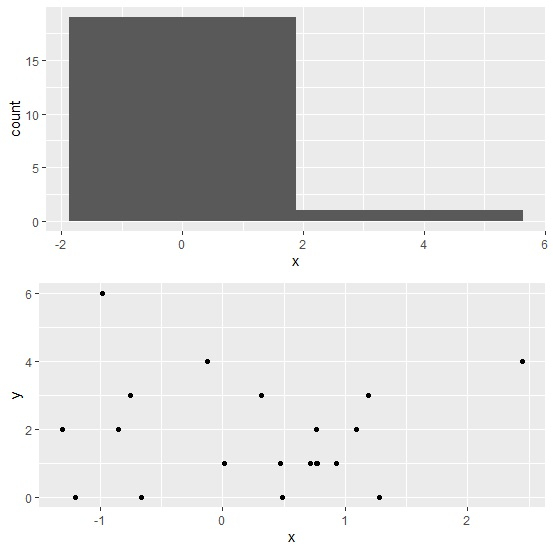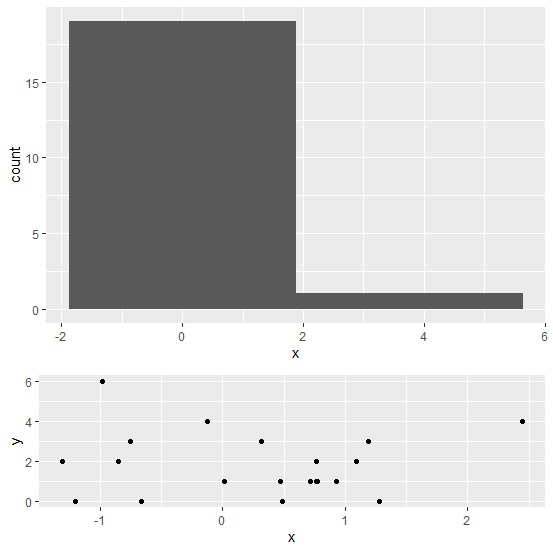# How to change the size of plots arranged using grid.arrange in R?

To change the size of plots arranged using grid.arrange, we can use heights argument. The heights argument will have a vector equal to the number of plots that we want to arrange inside grid.arrange. The size of the plots will vary depending on the values in this vector.

Consider the below data frame −

## Example

Live Demo

x<-rnorm(20)
y<-rpois(20,2)
df<-data.frame(x,y)
df

## Output

          x      y
1 2.44725895     4
2 -1.30716203    2
3 -0.98084721    6
4 0.31704022     3
5 1.09460383     2
6 0.76611276     1
7 0.93073818     1
8 -0.84915028    2
9 1.27882442     0
10 0.77428764    1
11 0.48511829    0
12 -1.20333353   0
13 -0.66665709   0
14 -0.12132914   4
15 0.46865269    1
16 0.71771117    1
17 0.76447810    2
18 0.01445573    1
19 -0.75449056   3
20 1.18946339    3

library(ggplot2)
p1<-ggplot(df,aes(x))+geom_histogram(bins=2)
p2<-ggplot(df,aes(x,y))+geom_point()

## Example

library(gridExtra)
grid.arrange(p1,p2)

## OutputArranging plots using grid.arrange with varying heights −

## Example

grid.arrange(p1,p2,heights=c(2,1))

## Output